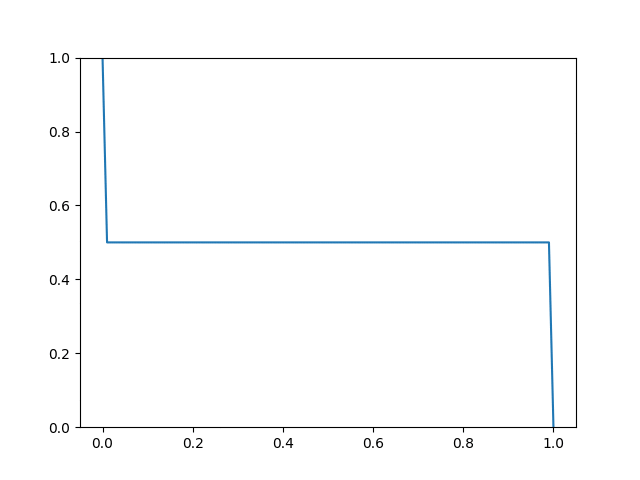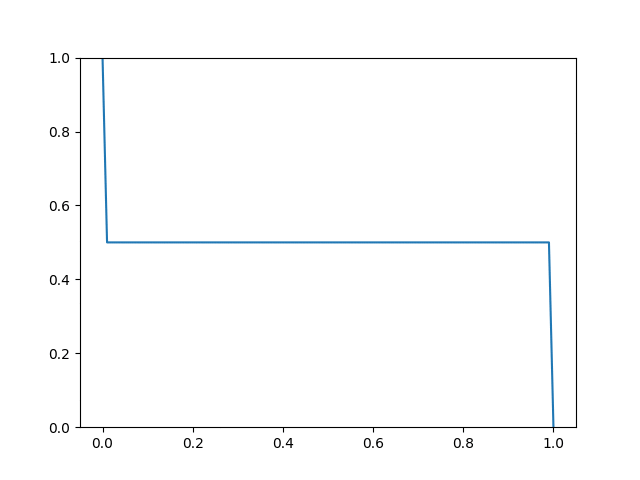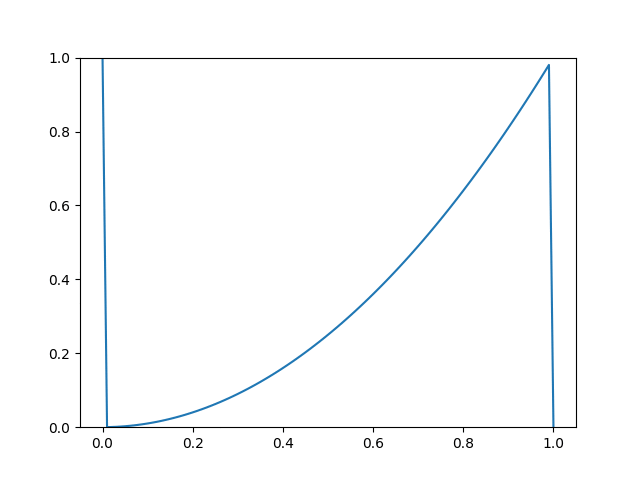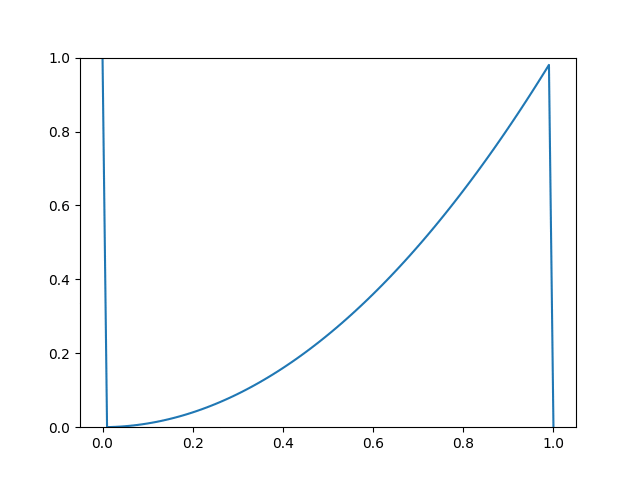Lately, I came to the realization that the process of thoroughly understanding a scientific theory or fact may be divided into three tiers:

• Concept (C)
• Math (M)
• Code (C)

A concept or idea of the approach is needed in order wrap your head around the thing you are trying to understand. This concept may be a very vague yet profound statement boiling down some essential scientific findings to a single sentence or a couple of sentences at most. Some scientists even argue that the shorter a scientific theory can be summarized, the more fundamental its nature can be understood. The same goes for good explanations of concepts: The simpler the better. Or, to put it like Albert Einstein:

“If you can’t explain it simply, you don’t understand it well enough.”

Take for instance the problem of heat conduction in materials. A very abstract concept of the conduction of heat may be the following statement: “Heat flows from hot areas to cold areas”. Already this simple statement allows us to make meaningful predictions about the future state of a system. If you touch a hot stove, you know that your hand will sooner or later become hot. You cannot make precise predictions about the concrete time span in which your hand will get or the concrete temperature your hand will have but you have a rough idea. Are more advanced concept to grasp may be the wave-particle-duality describing the alleged discrepancy between describing a particle as a wave and as a particle at the same time. Quantum mechanics allowed us to develop a new framework or concept in which a particle as both a wave and a particle at the same time. Having a good grasp of the concept of a theory is the first step in understanding this theory.

## Math

Mathematical equations describe the concept in the most abstract possible way. While the concept draws from your intuition and allows for a qualitative prediction of the future state of a system, mathematical equations allow us to precisely express quantitative properties of the system. In the context of heat conduction in materials, the 3-D heat-diffusion-equation allows us to make precise statements about the temperature u of a material as a function of space and time:

\begin{align*} \frac{\partial u}{\partial t} = \alpha \nabla^2 u = \alpha \Bigg( \frac{\partial^2 u}{\partial x^2} + \frac{\partial^2 u}{\partial y^2} + \frac{\partial^2 u}{\partial z^2}\Bigg) \end{align*}

The constant alpha denotes the thermal diffusivity of the material, which is defined as the thermal conductivity k over the product of the material density rho and the specific heat capacity $$c_p$$:

\begin{align*} \alpha = \frac{k}{c_p \rho} \end{align*}

Thinking about the math behind a concept allows you to deeply dive into the problem. It allows you to set the different parameters of the system into a relationship with another. In this example, you do not need to immediately see a possible solution to this differential equation. You might, however, understand that the spatial and temporal derivatives of the field quantity temperature are in a special relationship with one another, which is true at every point in space and at every moment in time.

Of course, other problems might have much more complex equations and might require a much higher mental workload in order for you to hold all the complex ideas in your head. Take, again, our wave-particle duality concept: The mathematical equation describing a quantum particle is a partial differential equation as well: The Schrödinger equation:

\begin{align*} i \hbar \frac{\partial \Psi}{\partial t} = - \frac{\hbar^2}{2 m} \nabla^2 \Psi + V \Psi \end{align*}

This equation describes the behavior of the wave function $$\Psi$$ of a quantum particle. While the Schrödinger equation is arguably more complex and difficult to grasp, it has the same fundamental properties as the heat equation: The time derivative of the function we try to obtain is in direct relationship to its second spatial derivative.

Pro-tip: Do not let yourself be intimidated by mathematical notation. It might sometimes look overwhelming but notation usually is only needed to write down an obvious statement in a water-proof fashion.

## Code

I lied to you in the last paragraph. You do not understand the math until you implemented it in code. Ok, this might be an overstatement but I heavily encourage you to implement your math in code, otherwise you might fall for the illusion of understanding your problem without actually and thoroughly understanding it.

Writing code is useful. First of all, it helps you to play around with your problem. Mathematical equations are great for expressing complex relationships between objects. However, seeing those relationships in action in your computer adds a whole new level to your understanding of the problem. It allows you to “experience” the mathematics behind the problem. It lets you play with parameters and see how the system state changes due to these differently chosen parameters.

And secondly, writing your problem in terms of code, lets you view your problem from different angles. It makes you think about the implications of possible solutions. Writing code also allows you to easily visualize your problem and the theory behind it. If you have a concise visualization of your problem, you can easily make a mental model of the system and its behavior. I deeply believe that anyone who understands a scientific theory and says that he does not have some kind of visualization of the problem in his mind does not really tell the truth.

Let us get back to our heat equation and implement a simple program to get a solution to the equation:

To make visualization easier, let us focus on one-dimensional heat conduction problems. This boils the differential equation down to the following form:

\begin{align*} \frac{\partial u}{\partial t} = \frac{k}{c_p \rho} \Bigg( \frac{\partial^2 u}{\partial x^2}\Bigg) \end{align*}

First of all, we need to specify a discrete representation of the continuous differential equation of heat conduction stated previously:

\begin{align*} \frac{u_i^{n+1} - u_i^{n}}{\Delta t} = \alpha \frac{u_{i+1}^{n} - 2 u_i^{n} + u_{i-1}^{n}}{2 \Delta x^2} \end{align*}

The originally continuous temperature u is discretized into discrete points in space and time. The subscripts i and the superscripts n denote the i-th spatial coordinate and n-th time step, respectively. The discrete approximation of the spatial derivative was obtained using the central differences approach while the discrete approximation of the temporal derivative was obtained using the (forward) Euler-method.

In order to find a solution, we solve the above equation for $$u_i^{n+1}$$ and iterate through all time steps in an outer loop and through all spatial points in an inner loop. We also have to apply initial conditions for $$u(x, t=0)$$ and boundary conditions for $$u(x=0,t)$$ and $$u(x=L,t)$$, while $$x=0$$ and $$x=L$$ denote the left and right ends of the 1-D simulation domain.

The code get a solution to this equation follows and is fairly straightforward and should be self explaining:

import numpy as np
import matplotlib.pyplot as plt
import time

L = 1.0 # length of 1-D heat-conducting object
Nx = 100 # number of spatial grid points
T = 10.0 # maximum time
Nt = 1000 # number of time steps
a = 0.005 # material proberty alpha

x = np.linspace(0, L, Nx+1)    # mesh points in space
dx = x - x

t = np.linspace(0, T, Nt+1)    # mesh points in time
dt = t - t

u   = np.zeros(Nx+1)           # unknown u at new time step
u_1 = np.zeros(Nx+1)           # u at the previous time step

# plotting boilerplate
fig = plt.figure()
ax.set_ylim([0,1])
li, = ax.plot(x, u)
ax.relim()
ax.autoscale_view(True,True,True)
fig.canvas.draw()
plt.show(block=False)

# definition of initial conditions
def initial(x):
return x**2

# Set initial condition u(x,0) = initial(x)
for i in range(0, Nx+1):
u_1[i] = initial(x[i])

# loop through every time step
for n in range(0, Nt):

# Compute u at inner grid points
for i in range(1, Nx):
u[i] = u_1[i] + a*dt/(dx*dx)*(u_1[i-1] - 2*u_1[i] + u_1[i+1])

# Appöy boundary conditions
u = 1.
u[Nx] = 0.

# Update u_1 before next step
u_1[:]= u

# plot every 10 time steps
if n % 10 == 0:
li.set_ydata(u)
fig.canvas.draw()
time.sleep(0.001)
plt.savefig('frames/' + str(n).zfill(3) + '.png')


The visualization of the solution to this simple differential equation should add a deeper understanding of the process of heat conduction through materials. The following three GIFS show the change in the material temperature over time. Boundary conditions of $$u(x=0,t) = 1.0$$ and $$u(x=1.0,t) = 0.0$$ are applied for all three setups.Slow heat conductionFast heat conductionFast heat conduction with different initial conditions

Playing around with the heat conduction parameters should make you develop a new intuition regarding the dynamical behavior of the system.

As a side-note: You might also encounter some numerical stability issues when selecting certain time step sizes:Numerical divergence of solution due to time step size

This problem is known as the Courant–Friedrichs–Lewy condition, which states that there is an upper limit to any time step size Delta t in finite differences schemes, which depends on the speed of information flow though the simulation domain and the length interval $$\Delta x$$.

Using the C-M-C approach, you should have developed an intuitive understanding of the whole problem. Going from an abstract concept you were able to understand the math behind the problem and were thus able to implement the equations in program code. Tinkering with parameters and visualizing your results allowed you to more deeply understand the problem and even find some numerical issues with the finite differences solution scheme.

Congrats, now you are a real problem-solver!

Let me know in the comments whether you think that the C-M-C approach can be viewed as a easy-to-follow guide to help you understand difficult problems and if you what approaches to improve your understanding of a topic you use in your daily scientific struggles.